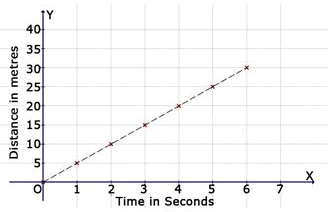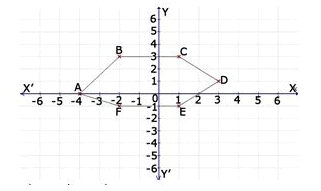Test: Introduction To Graphs- 1

# Test: Introduction To Graphs- 1

Test Description

## 10 Questions MCQ Test Mathematics (Maths) Class 8 | Test: Introduction To Graphs- 1

Test: Introduction To Graphs- 1 for Class 8 2023 is part of Mathematics (Maths) Class 8 preparation. The Test: Introduction To Graphs- 1 questions and answers have been prepared according to the Class 8 exam syllabus.The Test: Introduction To Graphs- 1 MCQs are made for Class 8 2023 Exam. Find important definitions, questions, notes, meanings, examples, exercises, MCQs and online tests for Test: Introduction To Graphs- 1 below.
Solutions of Test: Introduction To Graphs- 1 questions in English are available as part of our Mathematics (Maths) Class 8 for Class 8 & Test: Introduction To Graphs- 1 solutions in Hindi for Mathematics (Maths) Class 8 course. Download more important topics, notes, lectures and mock test series for Class 8 Exam by signing up for free. Attempt Test: Introduction To Graphs- 1 | 10 questions in 10 minutes | Mock test for Class 8 preparation | Free important questions MCQ to study Mathematics (Maths) Class 8 for Class 8 Exam | Download free PDF with solutions
 1 Crore+ students have signed up on EduRev. Have you?
Test: Introduction To Graphs- 1 - Question 1

### In which quadrant does the point P (-4, 1) lie?

Detailed Solution for Test: Introduction To Graphs- 1 - Question 1 It is because 2nd quadrant Xi axis consist of negative number and Y axis has positive numbers .i.e. (-,+)
Test: Introduction To Graphs- 1 - Question 2

### On which axis does the point (-1, 0) lie?

Detailed Solution for Test: Introduction To Graphs- 1 - Question 2

the point (-1, 0) lie on X axis as the Y value is 0 .

Test: Introduction To Graphs- 1 - Question 3

### Find the distance covered in 2 seconds.Detailed Solution for Test: Introduction To Graphs- 1 - Question 3 10m is the correct answer because in the graph at 2 seconds, the line is on 10m.
Test: Introduction To Graphs- 1 - Question 4

Find the distance covered in 5 seconds.Test: Introduction To Graphs- 1 - Question 5

Find the distance covered in 7 seconds.Test: Introduction To Graphs- 1 - Question 6

Find the coordinates of the point B from the graph.Detailed Solution for Test: Introduction To Graphs- 1 - Question 6

The point is -2 units from x-coordinate and 3 units from y coordinate, hence the coordinates of the point is (-2,3).

Test: Introduction To Graphs- 1 - Question 7

Find the distance covered in 6 seconds.Test: Introduction To Graphs- 1 - Question 8

Find the distance covered in 4 seconds.Test: Introduction To Graphs- 1 - Question 9

Find the coordinates of the point A from the graph.Test: Introduction To Graphs- 1 - Question 10

Find the distance covered in 3 seconds.Detailed Solution for Test: Introduction To Graphs- 1 - Question 10 As in this graph it shows that distance covered in 3 see second is 15 meter.

## Mathematics (Maths) Class 8

193 videos|360 docs|48 tests
 Use Code STAYHOME200 and get INR 200 additional OFF Use Coupon Code
Information about Test: Introduction To Graphs- 1 Page
In this test you can find the Exam questions for Test: Introduction To Graphs- 1 solved & explained in the simplest way possible. Besides giving Questions and answers for Test: Introduction To Graphs- 1, EduRev gives you an ample number of Online tests for practice

## Mathematics (Maths) Class 8

193 videos|360 docs|48 tests